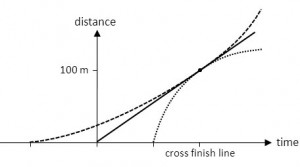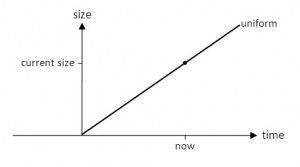# Graph the graph on the graph

I was creating a worksheet for our #astro101 class about the expansion of the Universe. If the Universe is expanding at a uniform rate, it’s about 14 billion years old. If the expansion is accelerating (decelerating), a little logic tells us the Universe must be older (younger) than 14 billion years.

I wrote the worksheet as a ranking task (“Rank the 3 models by expansion rate 1 billion years ago” and so on) using the great collection at UNL as a template. There’s also a nice graph that helps summarize the current, past and future expansion of the 3 models. This is the graph for my analogy of 3 runners, Connie (who runs at a constant rate), Alice (who accelerates) and Deena (who decelerates) practicing for a 100-metre race. The Universe version is identical except “distance” is “size of the Universe” and “cross finish line” is “now”.)Three runners cross the finish line at the same time and going the same speed. When did they start running?

I agonized (well, that’s a bit strong but you know what I mean) over getting the students to draw the 3 curves for the uniform, accelerating and decelerating Universes or getting them to identify and label the curves given in a diagram. Fortunately, we have nice set of learning goals for the course and one says, “You will be able to sketch different scenarios for the evolution of the size of the Universe, including when the Big Bang happened and the fate of the Universe.” That clearly told me to use “Sketch…” instead of “Label…”

Great. But is “sketch” the right verb? Soon, as a colleague and I started listing all the graphing nouns and verbs we use interchangeably, I realized once again that students most likely have many interpretations of these words. My “expert” interpretation is different than their “novice” interpretation of words like

• sketch
• draw
• graph (noun and verb)
• axes
• diagram
• figure
• plot (noun and verb)
• curve
• function

It’s not inconceivable that a student could be asked to “graph the graph on the graph” or “plot the plot on the plot”. Ay caramba!

In the end, I asked the students first to “write labels Connie, Alice and Deena next to each runner’s curve in the graph” (the one above). I figured that showed them the critical feature of the story, that all three runners crossed the line at the same time and going the same speed. Then later I asked

This graph shows the size of the Universe at each time for the uniform expansion model. Sketch the curves for the accelerating and decelerating universes. Remember that all curves must go through the current Universe  and all curves must have the same slope at that point because the slope is the Hubble Constant. Label the curves accelerating and decelerating.If the Universe expands at a uniform rate, right now is had its current size.

The students spent about 15 minutes on the worksheet. I’m happy to report that 103 of 115 (or 90%) of the students correctly chose C) older on this post-activity clicker question

If we discover the Universe is expanding at an accelerated rate, it means the Universe is

A) younger than 14 billion years
B) 14 billion years old
C) older than 14 billion years